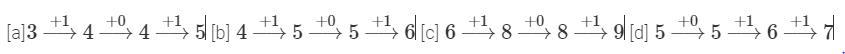Courses

# Olympiad Test: Patterns - 1

## 20 Questions MCQ Test Cyber Olympiad Class 4 | Olympiad Test: Patterns - 1

Description
This mock test of Olympiad Test: Patterns - 1 for Class 4 helps you for every Class 4 entrance exam. This contains 20 Multiple Choice Questions for Class 4 Olympiad Test: Patterns - 1 (mcq) to study with solutions a complete question bank. The solved questions answers in this Olympiad Test: Patterns - 1 quiz give you a good mix of easy questions and tough questions. Class 4 students definitely take this Olympiad Test: Patterns - 1 exercise for a better result in the exam. You can find other Olympiad Test: Patterns - 1 extra questions, long questions & short questions for Class 4 on EduRev as well by searching above.
QUESTION: 1

### Find the odd one out.

Solution:

D+1 = E, G + 1 = H, L + 1 = M but O – 1 = N.

QUESTION: 2

Solution:
QUESTION: 3

### Find the odd one out.

Solution:

R – 1 = Q, N – 1 = M, J – 1 = I but P+ 1 = Q.

QUESTION: 4

Find the odd one out.

Solution:

V + 1 = W, S + 1 = T, P + 1= Q but X+2 = Z.

QUESTION: 5

Find the odd one out.

Solution:
QUESTION: 6

Find the odd one out.

Solution:
QUESTION: 7

Find the odd one out.

Solution:
QUESTION: 8

Find the odd one out.

Solution:Hence, option [d] is the odd one.

QUESTION: 9

Find the odd one out.

Solution:
QUESTION: 10

Find the odd one out.

Solution:
QUESTION: 11

Find the odd one out.

Solution:
QUESTION: 12

Find the odd one out.

Solution:
QUESTION: 13

Find the odd one out.

Solution:
QUESTION: 14

Find the odd one out.

Solution:
QUESTION: 15

Find the odd one out.

Solution:
QUESTION: 16

Find the pair of letters that is most suited.
BE?AS

Solution:
QUESTION: 17

Find the pair of letters that is most suited.
BOT?URT

Solution:
QUESTION: 18

Find the pair of letters that is most suited.
SHAR?RINT

Solution:
QUESTION: 19

Find the pair of letters that is most suited.
CAME?IGHT

Solution:
QUESTION: 20

Find the pair of letters that is most suited.
HAN?ATE

Solution: